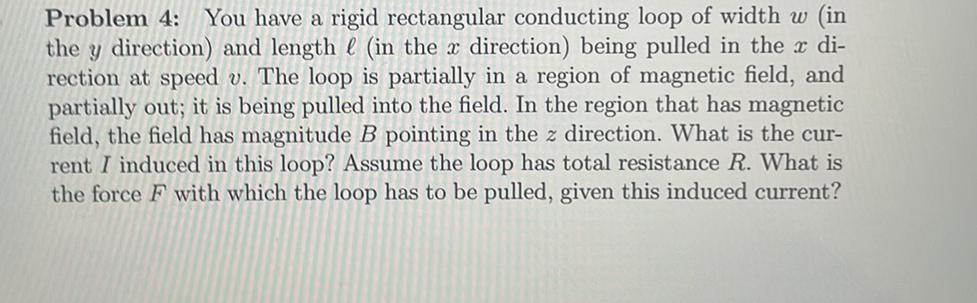Question:

# Problem 4: You have a rigid rectangular conducting loop of width w (in the y direction) and length l (in the x direction) beingProblem 4: You have a rigid rectangular conducting loop of width w (in the y direction) and length l (in the x direction) being pulled in the x di- rection at speed v. The loop is partially in a region of magnetic field, and partially out; it is being pulled into the field. In the region that has magnetic field, the field has magnitude B pointing in the z direction. What is the cur- rent I induced in this loop? Assume the loop has total resistance R. What is the force F with which the loop has to be pulled, given this induced current?